Espinosa, L., Brancheriau, L., Prieto, F., and Lasaygues, P. (2018). "Sensitivity of ultrasonic wave velocity estimation using the Christoffel equation for wood non-destructive characterization," BioRes. 13(1), 918-928.

#### Abstract

To perform a non-destructive evaluation of wood, the Christoffel equation is frequently used to describe the relationship between the ultrasonic wave velocity and the mechanical parameters. In the context of acoustical tomography imaging of standing trees, the key contribution of this numerical study is to determine the influence of mechanical parameters of the wood radial-tangential plane on the wave velocity computation using the Christoffel equation. Mechanical parameters from six species were selected. A sensitivity analysis was carried out by increasing and decreasing every parameter by a given percentage, and then by computing the variation of velocity for a set of wave direction of propagations. The evolution of the wave velocity, according to the direction of propagation, depended on the considered species; there was a difference between the softwoods and the hardwoods. The sensitivity analysis showed a bigger influence of the Young’s moduli, followed by the Poisson’s ratio, and finally by the shear modulus. However, these last two parameters cannot be neglected when using the Christoffel equation to solve the inverse problem of standing tree tomography. A proposed solution involves determining the propagation paths using the Young’s moduli as variables and then inversing the set of equations in accordance with the overall parameters.

Sensitivity of Ultrasonic Wave Velocity Estimation Using the Christoffel Equation for Wood Non-Destructive Characterization

Luis Espinosa,a,b,* Loïc Brancheriau,a Flavio Prieto,b and Philippe Lasaygues c

To perform a non-destructive evaluation of wood, the Christoffel equation is frequently used to describe the relationship between the ultrasonic wave velocity and the mechanical parameters. In the context of acoustical tomography imaging of standing trees, the key contribution of this numerical study is to determine the influence of mechanical parameters of the wood radial-tangential plane on the wave velocity computation using the Christoffel equation. Mechanical parameters from six species were selected. A sensitivity analysis was carried out by increasing and decreasing every parameter by a given percentage, and then by computing the variation of velocity for a set of wave direction of propagations. The evolution of the wave velocity, according to the direction of propagation, depended on the considered species; there was a difference between the softwoods and the hardwoods. The sensitivity analysis showed a bigger influence of the Young’s moduli, followed by the Poisson’s ratio, and finally by the shear modulus. However, these last two parameters cannot be neglected when using the Christoffel equation to solve the inverse problem of standing tree tomography. A proposed solution involves determining the propagation paths using the Young’s moduli as variables and then inversing the set of equations in accordance with the overall parameters.

Keywords: Wood; Ultrasonic; Wave propagation; Orthotropy; Christoffel equation

Contact information: a: CIRAD, UR BioWooEB, 73 Rue J.F. Breton, Montpellier, France; b: Dept. of Mechanical and Mechatronics Engineering, Universidad Nacional de Colombia, Carrera 45 N° 26-85, Bogotá, Colombia; c: Aix Marseille Univ, CNRS, Centrale Marseille, LMA, 4, Impasse Nikola TESLA, CS 40006, Marseille, France; *Corresponding author: lfespinosam@unal.edu.co

INTRODUCTION

Ultrasonic, non-destructive evaluation methods have been widely used for wood mechanical characterization and decay detection in trees (Saadat-Nia et al. 2011; Reinprecht and Hibký 2011; Hassan et al. 2013; Corigliano et al. 2017). These methods are based on the determination of the wave propagation velocity, as this parameter is directly related to the inner-wood mechanical properties (Ross 2015). Other wood parameters that affect wave propagation include the moisture content, grain orientation, anisotropy, viscoelastic behavior, and wood alterations or modifications (Beall 2002; Bucur 2006).

Wave motion in a homogeneous anisotropic elastic solid can be described by a set of partial differential equations. The Christoffel equation leads to a solution for this set of equations in the form of plane waves, relating the propagation velocity with the material elastic constants and the wave direction of propagation (Royer and Dieulesaint 2000).

Therefore, this relationship can be used to pass from a set of measured velocities to an estimation of the mechanical parameters, a procedure known as inverse problem (Bucur and Archer 1984; Castagnede and Sachse 1989; Bucur 2006; Dahmen et al. 2010; Longo et al. 2012; Gonçalves et al. 2014; Alves et al. 2015; Tallavo et al. 2017). For instance, ultrasonic goniometry relies on the principle of inverse problem to determine the elastic constants of the stiffness matrix for the characterization of different composite materials (Siva et al. 2005; Zhao et al. 2016), including wood (Preziosa 1982; Bachtiar et al. 2017). The mentioned procedure works by using small samples with cubic, prismatic, polyhedral geometry, or multifaceted discs, to determine the nine elastic constants of wood, by assuming the homogeneity of the specimens, and thus that the mechanical properties are constants within the specimens.

In the context of the acoustical tomography imaging of standing trees, the wave velocity values are determined for each local area (pixel of the resulting map) of the scanned cross section by solving an ill-conditioned inverse problem with a low number of acoustic measurements (Arciniegas et al. 2014). This problem is solved assuming that the transverse cross section of trees is quasi-isotropic. The hypothesis of isotropy blurs the image and makes it difficult to characterize the mechanical state of wood and the presence of a defect (Arciniegaset al. 2014; Espinosa et al. 2017). A way to overcome this problem is to consider the cross section of a standing tree as being cylindrically orthotropic in the process of inversion, such that the 4 elastic constants of wood for each pixel in the radial-tangential plane could be determined.

This work examined the sensitivity of the mechanical parameters in the computation of the compression wave velocity using the Christoffel equation, by means of a numerical study. First, published data were used to study the effect of anisotropy, according to the orientation of the wave front relative to the radial-tangential plane. Additionally, fluctuations in each value of mechanical parameters were introduced in the velocity computation according to the orientation of the wave front. The results made it possible to examine the consequences of proposing various simplified hypotheses based on an inversion process for standing tree tomography.

EXPERIMENTAL

Materials

Table 1 presents the tree species selected from previously published data (Forest Products Laboratory 2010). The species were chosen to cover a wide range of transverse anisotropy ratio, mechanical parameters, and density. ER/ET is the anisotropy ratio between the stiffnesses in the radial-tangential directions. The variation range of the anisotropy ratio is from 1.36 for Douglas fir to 2.30 for sweetgum.

The species in Table 1 are ranked according to the ratio ER/ET. The first half of this table corresponds to softwoods and the second half, to hardwoods. The radial (ER) and tangential (ET) Young’s modulus ranged between 909 MPa to 2118 MPa for ER, and between 511 MPa to 1128 MPa for ETGRT and νRT correspond to the shear modulus and the Poisson’s ratio. The shear modulus ranged between 36 MPa and 319 MPa, and the Poisson’s ratio ranged between 0.38 and 0.70. Density, noted as ρ, ranged from 448 to 706 kg/m3.

Table 1. Selected Species and Corresponding Mechanical Parameters. ER and ET: Young’s modulus in the radial and tangential directions, respectively, GRT: shear modulus, νRT: Poisson’s ratio, ρ: density. From published data (Forest Products Laboratory 2010)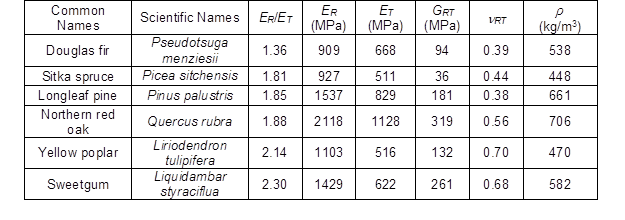Methods

At the macroscopic level, wood is considered an orthotropic material. The mechanical properties of wood change depending on the longitudinal axis (L) parallel to the fibers, the radial axis (R) orientated from the bark to the pith, and the tangential axis (T) tangent to the growth rings and perpendicular to the grain (Kollmann and Côté 1968). Combining the elastodynamic equation and the Hook’s law for a non-dispersive continuous and infinite media, a solution for the phase velocity V of a quasi-longitudinal plane wave is obtained using the Christoffel formulation in the wood radial-tangential (RT) plane (Royer and Dieulesaint 2000):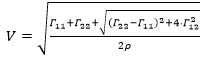(1)

In Eq. 1, is the wood density and the Christoffel coefficients Γ11Γ22 and Γ12 are a function of the elements of the stiffness matrix Cij (inverse of the compliance matrix S) for an orthotropic material (computed using the elastic constants of the RT plane ERETGRT, and νRT) and the direction of propagation indicated by the angle θ between the vector normal to the wave front and the radial direction,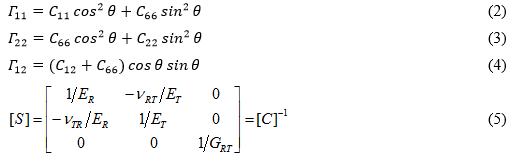A set of angles θ ranging from 0° (radial direction) to 90° (tangential direction) were used in Eq. 1 to evaluate the influence of the selected species (Table 1) on the wave velocity. The velocity values for each species were then computed by introducing a variation of ± 10% for the mechanical parameters (ERETGRT, and νRT) in order to evaluate the sensitivity of these parameters in Eq. 1. For example, the Young’s modulus in radial direction was increased as ERsup = 1.1 x ER and decreased as ERinf = 0.9 x ER.

The corresponding modified velocities values were named Vsup (velocity when the parameters were increased by 10%) and Vinf (velocity when the parameters were decreased by 10%). The variation of velocity (in percentage) for each parameter was obtained as follows: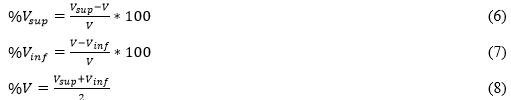RESULTS AND DISCUSSION

Figure 1 presents the wave velocity values depending on the angle θ for the selected species. Table 2 summarizes the minimum and maximum velocity values, ranging from 1065 m/s for spruce at θ = 59° to 1898 m/s for oak at θ = 0°.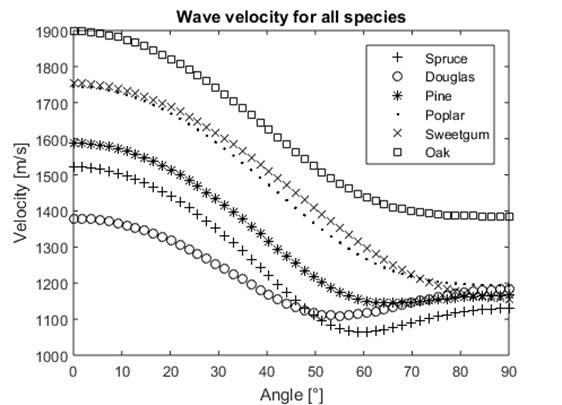Fig. 1. Velocity values for all species in function of the angle between the vector normal to the wave front and the radial direction

The relationship between the wave velocity and the direction of propagation (angle θ) is a direct consequence of the wood anisotropy in the RT plane. For all species, higher velocities were obtained in the radial direction (θ = 0°) due to the fact that this direction is stiffer than the tangential direction. The anisotropy between Eand ET can be approached by referring to the cellular microstructure of wood, which consists mainly of hollow tubular cells leading to an approximated honeycomb structure (Gillis 1972; Kahle and Woodhouse 1994). From this approach, several aspects have been linked to the anisotropic behavior of wood. First, the effect of the cell geometry: the cell walls are highly aligned in the radial direction, while the tangential direction follows an irregular pattern (Kahle and Woodhouse 1994), resulting in a higher Young’s modulus in the radial direction. Second, the mechanical properties change within the annual growth rings. Earlywood exhibits a marked anisotropic behavior (with large thin-walled cells aligned in the radial direction), while latewood exhibits a roughly isotropic behavior (with smaller and thicker-walled cells) (Boutelje 1962). Therefore, the proportion between earlywood and latewood affects the relationship between the radial-tangential moduli. Third, the presence of the ray cells reinforces the radial direction, depending on the width of the rays, height, and area fraction (Burgert et al. 2001). For hardwoods, an additional factor to be considered is the vessel distribution, with higher ER/ET ratios for diffuse porous species, such as yellow poplar, than ring porous species, such as oak (Beery et al. 1983).

Table 2. Maximum (Vmax) and Minimum (Vmin) Velocity Values with their Associated Angles (θ)Differences between maximum and minimum velocities were higher for hardwoods, as they presented a higher anisotropy ratio (difference of 596 m/s for sweetgum). In contrast, lower velocity differences were found in the trees with a lower anisotropy ratio (270 m/s for Douglas fir). For hardwoods, the minimum values of velocity were found in the pure tangential direction (θ = 90°). For softwoods, the minimum values were not found directly in the tangential direction, but in an angle ranging from 55° to 66° (Fig. 1 and Table 2). This angle depends mainly on GRT and νRT parameters, as they affect the off-diagonal parameter Γ12 in the Christoffel’s equation. The Γ12 coefficient has a higher influence on the velocity computation when the terms Γ11 and Γ22 are equal (the term inside the inner root square will only depend on Γ12), which occurred for angles ranging from 50° to 60°.

Table 3. Sensitivity Values Obtained with a Variation of 10% for Each Mechanical Parameter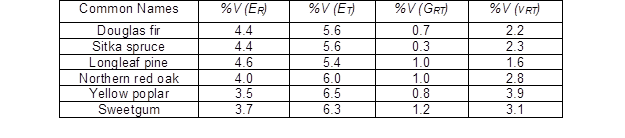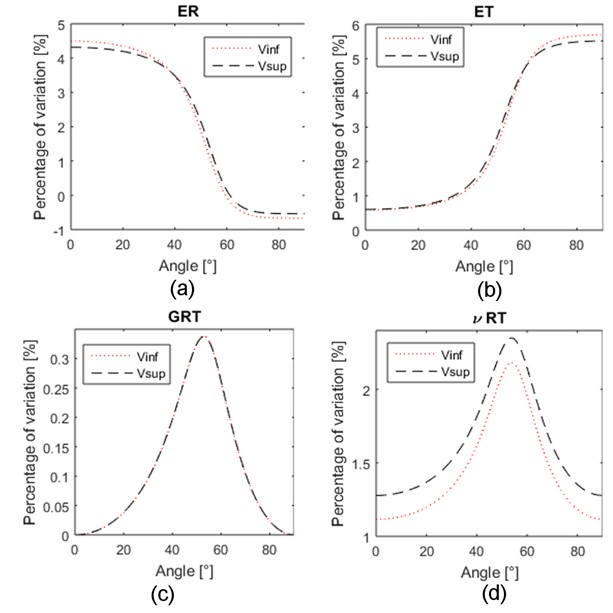Fig. 2. Velocity variations (in percentage) induced by changing each mechanical parameter for Sitka spruce according to the angle between the vector normal to the wave front and the radial direction (a) Radial Young modulus, (b) Tangential Young modulus, (c) Radial-Tangential shear modulus, and (d) Radial-Tangential Poisson coefficient

Each mechanical parameter was increased and decreased by 10%, and the velocity values were computed using Eq. 1. Table 3 showcases the maximums of the velocity variations (Eq. 7) after changing each parameter. For example, the variations of velocity values (Eqs. 5 and 6) for Sitka spruce are shown in Fig. 2. The velocity variation increased as the angle approached 0° when ER was altered (maximum variation of circa 4%, Table 3). On the contrary, the variation was at its maximum when the angle reached 90° for ET (maximum variation of 6%, Table 3). This was explained by the fact that the C11 element is predominant in Eq. 1 (axis 1 is associated with the radial direction) when the angle θ approaches to 0°. The same reasoning can be applied to the element C22 when the angle θ tends to 90° (tangential direction). GRT presented the lowest variation with a maximum of 0.34%, at an angle of 53° for Spruce in Fig. 2 (overall variation of 0.8%, Table 3). The maximum variation was found in angles ranging from 49° to 58° when GRT and νRT were changed to be almost equal for both parameters in each species (variation of 2.7% for νRT, Table 3). These angles increased as the ratio of anisotropy (ER/ET) increased (49° for Douglas fir and 58° for sweetgum). This phenomenon was already explained by the effect of the off-diagonal parameter Γ12 in the Christoffel’s equation. The velocity variations for changes in ERET, and νRT did not reach zero, as it did for GRT. This was explained by the fact that the velocity is computed using the stiffness constants Cij, which was modified by ERET, and νRT. Only the stiffness constant C66 was affected solely by GRT.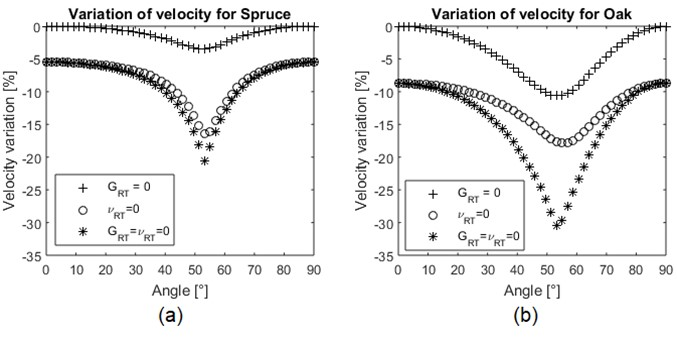Fig. 3. Velocity variation (in percentage) by setting νRT and GRT parameters to zero for (a) spruce and (b) oak species

Velocity values were more affected by the ET and ER parameters than by νRT and GRT. The order of influence, from biggest to smallest was ETERνRT, and GRT with a maximum variation of 5.9%, 4.1%, 2.7% and 0.8%, respectively. Each of the parameters was associated with an initial variation of 10%. Variations such as 4% and 6% on the velocity measurement for wood testing, within the same species, has been previously reported (Bucur 2006; Chauhan and Kumar 2014); thus, the influence on the velocity for a variation in the mechanical parameters of 10% is noteworthy. Figure 4 shows the variation of velocity %V divided by a variation in the mechanical parameters λ, with λ ranging between 10% to 50%. The relationship between these variations was not linear for ETER and νRT.

Considering the inverse problem in the case of standing tree acoustical tomography imaging, when passing from the velocity value to the mechanical parameters, the initial problem counts for 5 parameters (4 elastic parameters and the density) associated with each pixel of the tomogram. Bearing in mind the low sensitivity of νRT and GRT in Eq. 1, these two parameters would be determined with a low accuracy. A first approximation would be to set these two parameters to zero to find an initial solution, only for the two Young’s moduli, and then to use this solution to attempt again the inversion, but this time with all variables. To establish the velocity variation in this case, the νRT and GRT parameters were set to zero, and the corresponding velocity was compared to the velocity obtained using all the parameters. Figure 3 presents the variations obtained for spruce and oak species. As expected, a higher variation of velocity was obtained when νRT was nil compared to GRT (Fig. 3). When both parameters were nil, the variation of velocity was maximized for angles rating between 50° to 60°. However, even when the sensitivity of νRT and GRT on the velocity computation was low compared to the Young’s moduli, the maximum velocity variation was circa -20% for spruce and -30% for northern red oak (Fig. 3). Thus, it was concluded that νRT and GRT cannot be neglected, even in first approximations, for Eq. 1.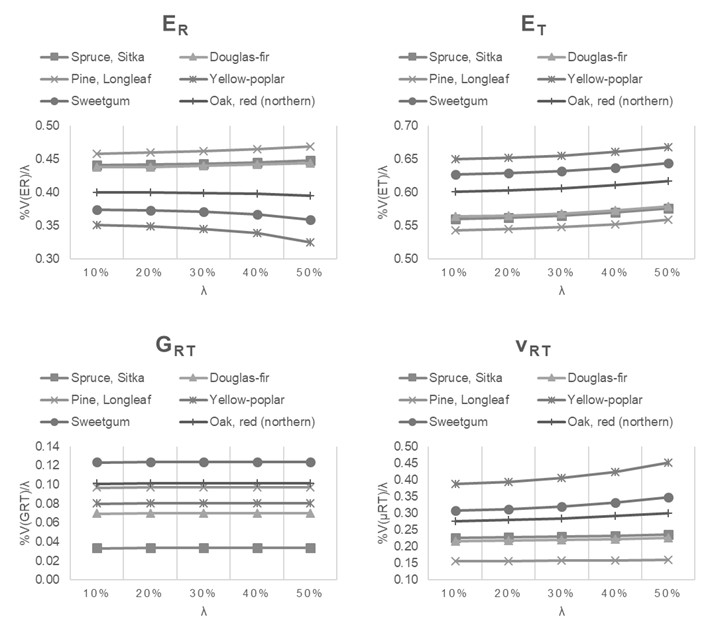Fig. 4. Velocity variation divided by the variation λ in the mechanical parameters, with λ ranging between 10% to 50%.

When taking into account the anisotropy of wood in the transverse cross section of a tree, the propagation paths of acoustic waves are curved, and not straight rays as they are for an isotropic material (Espinosa et al. 2017). As a result, the notion of wave velocity (considered as an intrinsic parameter of the material) associated with one pixel of the tomogram has no sense for anisotropic material, because the velocity depends on the direction of propagation. The only intrinsic parameters to be considered should be the elastic parameters and the density (5 intrinsic parameters). Under consideration that the knowledge on the specific stiffness (stiffness matrix divided by the density) is sufficient to allow for a tree health assessment, the number of unknowns for each pixel is reduced to four. In this case, the inversion process will lead to 4 tomograms associated to the 4 parameters. Since trajectories are not known a priori, an optimization procedure is required to modify iteratively ray paths, to minimize a functional of the time-of-flight. Next, for each pixel in every trajectory, the corresponding slowness (or velocity) value should be used to obtain the mechanical parameters, by means of the Christoffel formulation. A simplified hypothesis consisting in canceling certain unknowns cannot be used, but νRT and GRT have a low sensitivity in Eq. 1, and can be set as constants (published values). One solution is then to find, first the propagation paths by the use of an optimization method considering νRT and GRT as constants (which is to identify the anisotropy ratio ER/ET for each pixel). Then secondly, it is to write the set of independent equations and solve them with the constraint that 4 local equations define each pixel (with 4 unknowns).

CONCLUSIONS

1. The anisotropy of wood in the radial-tangential plane directly influences wave velocity, depending on the direction of propagation. The evolution of wave velocity according to the direction of propagation depends on the considered species, with a difference between softwoods and hardwoods. The radial direction, θ = 0°, corresponded to the fastest wave velocity. The shear modulus and Poisson’s ratio determined the angle for minimum velocity of softwoods, ranging from 55° to 66°. For hardwoods, the minimum velocity was in the tangential direction (θ = 90°).
2. From the sensitivity analysis of the Christoffel equation, it was found that the order of influence of the mechanical parameters on the velocity variation, from largest to smallest was: ETERνRT, and GRT. Considering an initial variation of 10% for each parameter, the maximum of the resulting velocity variations was 7 times higher for ET than for GRT. Young’s moduli influence was maximized when the direction of propagation was close to the tangential or radial axis. Poisson’s ratio and shear modulus influences were maximized in directions ranging from 50° to 60°.
3. Even if the influence of the Poisson’s ratio and shear modulus are low, the νRT and GRT parameters cannot be neglected in the Christoffel equation to solve the inverse problem of standing tree tomography. Thus, a proposed solution is to determine the propagations paths, in a first step, by setting the last two parameters as constants. The set of equations can then be solved, in a second step, under the consideration of the overall parameters with the addition of local equations in order to determine the unknowns for each pixel of the tomogram.

ACKNOWLEDGMENTS

This work was carried out in the framework of a project (C16A01) funded by the ECOS Nord program and is a part of Luis Espinosa’s Ph.D. thesis (grant from COLCIENCIAS – Departamento Administrativo de Ciencia, Tecnología e Innovación, Colombia).

REFERENCES CITED

Alves, R. C., Mantilla, J. N. R., Bremer, C. F., and Carrasco, E. V. M. (2015). “Application of acoustic tomography and ultrasonic waves to estimate stiffness constants of muiracatiara Brazilian wood,” BioResources 10(1), 1845-1856. DOI: 10.15376/biores.10.1.1845-1856

Arciniegas, A., Prieto, F., Brancheriau, L., and Lasaygues, P. (2014). “Acoustic and ultrasonic tomography of standing trees: Literature review and signal processing open problems,” Trees – Structure and Function 28(6), 1559-1567. DOI: 10.1007/s00468-014-1062-6

Bachtiar, E., Sanabria, S., Mittig, J., and Niemz, P. (2017). “Moisture-dependent elastic characteristics of walnut and cherry wood by means of mechanical and ultrasonic test incorporating three different ultrasound data evaluation techniques,” Wood Science and Technology 51(1), 47-67. DOI: 10.1007/s00226-016-0851-z

Beall, F. C. (2002). “Overview of the use of ultrasonic technologies in research on wood properties,” Wood Science and Technology 36(3), 197-212. DOI: 10.1007/s00226-002-0138-4

Beery, W. H., Ifju, G., and McLain, T. E. (1983). “Quantitative wood anatomy-relating anatomy to transverse tensile strength,” Wood and Fiber Science 15(4), 395-407.

Boutelje, J. B. (1962). “The relationship of structure to transverse anisotropy in wood with reference to shrinkage and elasticity,” Holzforschung 16(2), 33-46. DOI: 10.1515/hfsg.1962.16.2.33

Bucur, V., and Archer, R. R. (1984). “Elastic constants for wood by an ultrasonic method,” Wood Science and Technology 18, 255-265. DOI: 10.1007/BF00353361

Bucur, V. (2006). “Theory of and experimental methods for the acoustic characterization of wood” in: Acoustics of Wood, Springer-Verlag, Berlin, pp. 39-104.

Burgert, I., Bernasconi, A., Niklas, K. J., and Eckstein, D. (2001). “The influence of rays on the transverse elastic anisotropy in green wood of deciduous trees,” Holzforschung 55(5), 449-454. DOI: 10.1515/HF.2001.074

Castagnede, B., and Sachse, W. (1989). “Optimized determination of elastic constants of anisotropic solids from wavespeed measurements,” in: Review of Progress in Quantitative Nondestructive Evaluation, Springer, New York, NY, USA.

Chauhan, S., and Kumar, A. N. (2014). “Assessment of variability in morphological and wood quality traits in Melia dubia Cav. for selection of superior trees,” Journal of the Indian Academy of Wood Science 11(1), 25-32. DOI: 10.1007/s13196-014-0113-3

Corigliano, P., Crupi, V., Epasto, G., Guglielmino, E., Maugeri, N., and Marinò, A. (2017). “Experimental and theoretical analyses of Iroko wood laminates,” Composites Part B: Engineering 112, 251-264. DOI: 10.1016/j.compositesb.2016.12.049

Dahmen, S., Ketata, H., Ben Ghozlen, M. H., and Hosten, B. (2010). “Elastic constants measurement of anisotropic Olivier wood plates using air-coupled transducers generated lamb wave and ultrasonic bulk wave,” Ultrasonics 50(4-5), 502-507. DOI: 10.1016/j.ultras.2009.10.014

Espinosa, L., Prieto, F., and Brancheriau, L. (2017). “Ultrasonic imaging for non-destructive evaluation of standing trees: Effect of anisotropy on image reconstruction,” in: Thirteenth International Conference on Quality Control by Artificial Vision 2017, 1033808, Tokyo, Japan. DOI: 10.1117/12.2266757

Forest Products Laboratory (2010). Wood Handbook – Wood as an Engineering Material (General Technical Report FPL–GTR–190), U. S. Department of Agriculture Forest Products Laboratory, Madison, WI.

Gillis, P. P. (1972). “Orthotropic elastic constants of wood,” Wood Science and Technology, 6(2), 138-156. DOI: 10.1007/BF00350827

Gonçalves, R., Trinca, A. J., and Pellis, B. P. (2014). “Elastic constants of wood determined by ultrasound using three geometries of specimens,” Wood Science and Technology 48(2), 269-287. DOI: 10.1007/s00226-013-0598-8

Hassan, K. T. S., Horáček, P., and Tippner, J. (2013). “Evaluation of stiffness and strength of scots pine wood using resonance frequency and ultrasonic techniques,” BioResources 8(2), 1634-1645. DOI: 10.15376/biores.8.2.1634-1645

Kahle, E., and Woodhouse, J. (1994). “The influence of cell geometry on the elasticity of softwood,” Journal of Materials Science 29(5), 1250-1259. DOI: 10.1007/BF00975072

Kollmann, F. F., and Côté, W. A. (1968). “The structure of wood and the wood cell wall,” in: Principles of Wood Science and Technology: I Solid Wood, Springer-Verlag, Berlin, Germany, pp. 1 – 54.

Longo, R., Delaunay, T., Laux, D., El Mouridi, M., Arnould, O., and Le Clézio, E. (2012). “Wood elastic characterization from a single sample by resonant ultrasound spectroscopy,” Ultrasonics 52(8), 971-974. DOI: 10.1016/j.ultras.2012.08.006

Preziosa, C. (1982). Method for Determining the Elastic Constants of Wood Material by Using Ultrasound, Ph.D. Dissertation, University of Orleans, Orleans, France.

Reinprecht, L., and Hibký, M. (2011). “The type and degree of decay in spruce wood analyzed by the ultrasonic method in three anatomical directions,” BioResources 6(4), 4953-4968. DOI: 10.15376/biores.6.4.4953-4968

Ross, Robert J. (Ed.) (2015). Nondestructive Evaluation of Wood: Second Edition (General Technical Report FPL-GTR-238), U.S. Department of Agriculture Forest Products Laboratory, Madison, WI.

Royer, D., and Dieulesaint, E. (2000). “Plane waves in crystals,” in: Elastic Waves in Solids, Springer, Berlin, pp. 171-260.

Saadat-Nia, M., Brancheriau, L., Gallet, P., Enayati, A. A., Pourtahmasi, K., and Honavar, F. (2011). “Ultrasonic wave parameter changes during propagation through poplar and spruce reaction wood,” BioResources 6(2), 1172-1185. DOI: 10.15376/biores.6.2.1172-1185

Siva Shashidhara Reddy, S., Balasubramaniam, K., Krishnamurthy, C. V., and Shankar, M. (2005). “Ultrasonic goniometry immersion techniques for the measurement of elastic moduli,” Composite Structures, 67(1), 3-17. DOI: 10.1016/j.compstruct.2004.01.008

Tallavo, F., Cascante, G., and Pandey, M. D. (2017). “Experimental verification of an orthotropic finite element model for numerical simulations of ultrasonic testing of wood poles,” European Journal of Wood and Wood Products 75(4), 543-551. DOI: 10.1007/s00107-016-1065-7

Zhao, J., Qiu, J., and Ji, H. (2016). “Reconstruction of the nine stiffness coefficients of composites using a laser generation based imaging method,” Composites Science and Technology126, 27-34. DOI: 10.1016/j.compscitech.2016.02.001

Article submitted: August 30, 2017; Peer review completed: October 29, 2017; Revised version received and accepted: November 28, 2017; Published: December 6, 2017.

DOI: 10.15376/biores.13.1.918-928